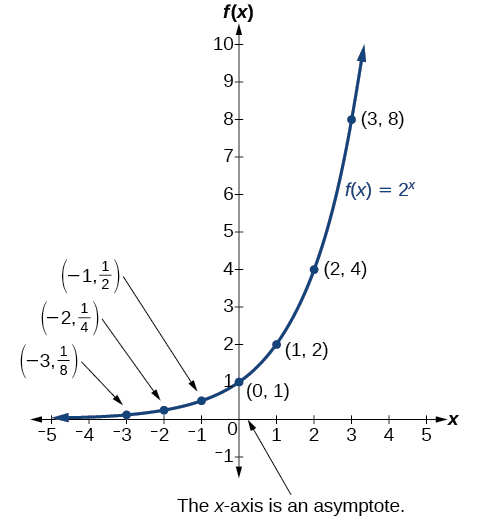# 4.2 Graphs of exponential functions

 Page 1 / 6
• Graph exponential functions.
• Graph exponential functions using transformations.

As we discussed in the previous section, exponential functions are used for many real-world applications such as finance, forensics, computer science, and most of the life sciences. Working with an equation that describes a real-world situation gives us a method for making predictions. Most of the time, however, the equation itself is not enough. We learn a lot about things by seeing their pictorial representations, and that is exactly why graphing exponential equations is a powerful tool. It gives us another layer of insight for predicting future events.

## Graphing exponential functions

Before we begin graphing, it is helpful to review the behavior of exponential growth. Recall the table of values for a function of the form $\text{\hspace{0.17em}}f\left(x\right)={b}^{x}\text{\hspace{0.17em}}$ whose base is greater than one. We’ll use the function $\text{\hspace{0.17em}}f\left(x\right)={2}^{x}.\text{\hspace{0.17em}}$ Observe how the output values in [link] change as the input increases by $\text{\hspace{0.17em}}1.$

 $x$ $-3$ $-2$ $-1$ $0$ $1$ $2$ $3$ $f\left(x\right)={2}^{x}$ $\frac{1}{8}$ $\frac{1}{4}$ $\frac{1}{2}$ $1$ $2$ $4$ $8$

Each output value is the product of the previous output and the base, $\text{\hspace{0.17em}}2.\text{\hspace{0.17em}}$ We call the base $\text{\hspace{0.17em}}2\text{\hspace{0.17em}}$ the constant ratio . In fact, for any exponential function with the form $\text{\hspace{0.17em}}f\left(x\right)=a{b}^{x},$ $\text{\hspace{0.17em}}b\text{\hspace{0.17em}}$ is the constant ratio of the function. This means that as the input increases by 1, the output value will be the product of the base and the previous output, regardless of the value of $\text{\hspace{0.17em}}a.$

Notice from the table that

• the output values are positive for all values of $x;$
• as $\text{\hspace{0.17em}}x\text{\hspace{0.17em}}$ increases, the output values increase without bound; and
• as $\text{\hspace{0.17em}}x\text{\hspace{0.17em}}$ decreases, the output values grow smaller, approaching zero.

[link] shows the exponential growth function $\text{\hspace{0.17em}}f\left(x\right)={2}^{x}.$Notice that the graph gets close to the x -axis, but never touches it.

The domain of $\text{\hspace{0.17em}}f\left(x\right)={2}^{x}\text{\hspace{0.17em}}$ is all real numbers, the range is $\text{\hspace{0.17em}}\left(0,\infty \right),$ and the horizontal asymptote is $\text{\hspace{0.17em}}y=0.$

To get a sense of the behavior of exponential decay , we can create a table of values for a function of the form $\text{\hspace{0.17em}}f\left(x\right)={b}^{x}\text{\hspace{0.17em}}$ whose base is between zero and one. We’ll use the function $\text{\hspace{0.17em}}g\left(x\right)={\left(\frac{1}{2}\right)}^{x}.\text{\hspace{0.17em}}$ Observe how the output values in [link] change as the input increases by $\text{\hspace{0.17em}}1.$

 $x$ $-3$ $-2$ $-1$ $0$ $1$ $2$ $3$ $g\left(x\right)=\left(\frac{1}{2}{\right)}^{x}$ $8$ $4$ $2$ $1$ $\frac{1}{2}$ $\frac{1}{4}$ $\frac{1}{8}$

Again, because the input is increasing by 1, each output value is the product of the previous output and the base, or constant ratio $\text{\hspace{0.17em}}\frac{1}{2}.$

Notice from the table that

• the output values are positive for all values of $\text{\hspace{0.17em}}x;$
• as $\text{\hspace{0.17em}}x\text{\hspace{0.17em}}$ increases, the output values grow smaller, approaching zero; and
• as $\text{\hspace{0.17em}}x\text{\hspace{0.17em}}$ decreases, the output values grow without bound.

[link] shows the exponential decay function, $\text{\hspace{0.17em}}g\left(x\right)={\left(\frac{1}{2}\right)}^{x}.$

The domain of $\text{\hspace{0.17em}}g\left(x\right)={\left(\frac{1}{2}\right)}^{x}\text{\hspace{0.17em}}$ is all real numbers, the range is $\text{\hspace{0.17em}}\left(0,\infty \right),$ and the horizontal asymptote is $\text{\hspace{0.17em}}y=0.$

## Characteristics of the graph of the parent function f ( x ) = b x

An exponential function with the form $\text{\hspace{0.17em}}f\left(x\right)={b}^{x},$ $\text{\hspace{0.17em}}b>0,$ $\text{\hspace{0.17em}}b\ne 1,$ has these characteristics:

• one-to-one function
• horizontal asymptote: $\text{\hspace{0.17em}}y=0$
• domain:
• range: $\text{\hspace{0.17em}}\left(0,\infty \right)$
• x- intercept: none
• y- intercept: $\text{\hspace{0.17em}}\left(0,1\right)\text{\hspace{0.17em}}$
• increasing if $\text{\hspace{0.17em}}b>1$
• decreasing if $\text{\hspace{0.17em}}b<1$

[link] compares the graphs of exponential growth    and decay functions.

Given an exponential function of the form $\text{\hspace{0.17em}}f\left(x\right)={b}^{x},$ graph the function.

1. Create a table of points.
2. Plot at least $\text{\hspace{0.17em}}3\text{\hspace{0.17em}}$ point from the table, including the y -intercept $\text{\hspace{0.17em}}\left(0,1\right).$
3. Draw a smooth curve through the points.
4. State the domain, $\text{\hspace{0.17em}}\left(-\infty ,\infty \right),$ the range, $\text{\hspace{0.17em}}\left(0,\infty \right),$ and the horizontal asymptote, $\text{\hspace{0.17em}}y=0.$

what's a domain
The set of all values you can use as input into a function su h that the output each time will be defined, meaningful and real.
Spiro
how fast can i understand functions without much difficulty
what is set?
a colony of bacteria is growing exponentially doubling in size every 100 minutes. how much minutes will it take for the colony of bacteria to triple in size
I got 300 minutes. is it right?
Patience
no. should be about 150 minutes.
Jason
It should be 158.5 minutes.
Mr
ok, thanks
Patience
100•3=300 300=50•2^x 6=2^x x=log_2(6) =2.5849625 so, 300=50•2^2.5849625 and, so, the # of bacteria will double every (100•2.5849625) = 258.49625 minutes
Thomas
what is the importance knowing the graph of circular functions?
can get some help basic precalculus
What do you need help with?
Andrew
how to convert general to standard form with not perfect trinomial
can get some help inverse function
ismail
Rectangle coordinate
how to find for x
it depends on the equation
Robert
yeah, it does. why do we attempt to gain all of them one side or the other?
Melissa
whats a domain
The domain of a function is the set of all input on which the function is defined. For example all real numbers are the Domain of any Polynomial function.
Spiro
Spiro; thanks for putting it out there like that, 😁
Melissa
foci (–7,–17) and (–7,17), the absolute value of the differenceof the distances of any point from the foci is 24.
difference between calculus and pre calculus?
give me an example of a problem so that I can practice answering
x³+y³+z³=42
Robert
dont forget the cube in each variable ;)
Robert
of she solves that, well ... then she has a lot of computational force under her command ....
Walter
what is a function?
I want to learn about the law of exponent

#### Get Jobilize Job Search Mobile App in your pocket Now!ByBy OpenStaxBy John GabrieliBy Jonathan LongBy Brooke DelaneyBy Megan EarhartBy Frank LevyBy P. Wynn NormanBy OpenStaxBy OpenStaxBy OpenStax